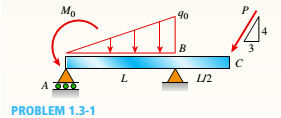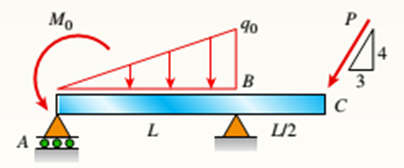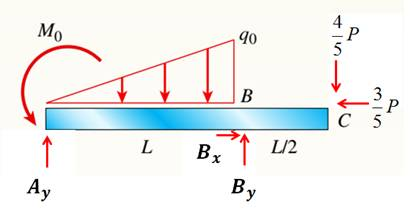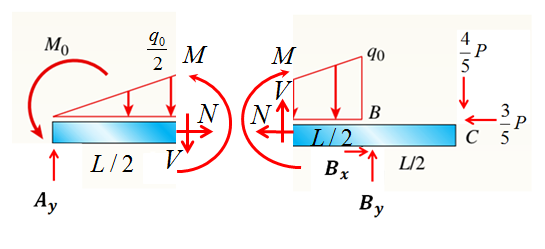# Find support reactions at A and B and then calculate the axial force N. shear force J and bending moment M at mid-span of AB. Let L = 14 ft. q 0 = 12 lb/ft. P = 50 lb. and = 300 lb-ft.BuyFind

### Mechanics of Materials (MindTap Co...

9th Edition
Barry J. Goodno + 1 other
Publisher: Cengage Learning
ISBN: 9781337093347BuyFind

### Mechanics of Materials (MindTap Co...

9th Edition
Barry J. Goodno + 1 other
Publisher: Cengage Learning
ISBN: 9781337093347
Chapter 1, Problem 1.3.1P
Textbook Problem

## Find support reactions at A and B and then calculate the axial force N. shear force J and bending moment M at mid-span of AB. Let L = 14 ft. q0 = 12 lb/ft. P = 50 lb. and = 300 lb-ft.Expert Solution
To determine

You need to calculate support reactions at Aand B; and then find axial force N, shear force Vand bending moment Mat mid span of the ABin the figure given below.## Answer to Problem 1.3.1P

The external reaction forces are Bx=30lb, By=94.57lb, Ay=29.4lb and internal reaction forces are axial force N=0, shear force V=8.42lb and moment M=143.06lb-ft.

### Explanation of Solution

Given Information:

You have a beam with different types of loading as shown in figure below.Figure 1 It is given that, L=14ft, q0=12lb/ft, P=50lb, and M0=300lb-ft.

Calculation:

To find external support reaction forces, draw free body diagram as shown in figure below.Figure 2: Free body diagram for external reactions Take equilibrium in xdirection (horizontal direction),

Fx=0Bx=35P=35×50 lb=30 lb

Take equilibrium of moments about point A,

MA=0M0+ByL=12q0L2L3+45P(L+L2)By=1L(M0+12q0L2L3+45P(L+L2))By=114(300+12×12×14×2×143+45×50×(14+142))By=94.57 lb

Take equilibrium in ydirection (vertical direction),

Fy=0Ay+By=45P+12q0LAy=By+45P+12q0LAy=94.57+45×50+12×12×14Ay=29.4 lb

To find internal forces at mid span of AB, draw the following free body diagram.Figure 3 Free body diagram for internal forces Now take left hand side free body diagram.

Take equilibrium in xdirection (horizontal direction),

Fx=0N=0

Take equilibrium in ydirection (vertical direction),

Fy=0Ay=V+12q02L2V=Ay12q02L2V=29.412×122×142V=8.42 lb

Take equilibrium of moments about point A,

MA=0M0+M=12q0L42L3×2+VL2M=M0+12q0L42L3×2+VL2M=300+12×12×144×2×143×2+8.42×142M=143.06 lb-ft

Conclusion:

Thus the external reaction forces are Bx=30lb, By=94.57lb, Ay=29.4lb and internal reaction forces are axial force N=0, shear force V=8.42lb and moment M=143.06lb-ft.

### Want to see more full solutions like this?

Subscribe now to access step-by-step solutions to millions of textbook problems written by subject matter experts!

### Want to see more full solutions like this?

Subscribe now to access step-by-step solutions to millions of textbook problems written by subject matter experts!© 2021 bartleby. All Rights Reserved.